# Water salinity from density equation

Jump to: navigation, search

## Water salinity from density equation

Handy equation to get the formation water (brine) salinity from the density at standard conditions obtained by the data regression with a stage line.

## Math and Physics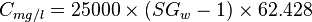$C_{mg/l} = 25000 \times (SG_w - 1) \times 62.428$

## Example. Calculating water salinity from density

Example source 

### Input data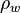$\rho_w$ = 65.4 lbm/ft3

Calculate water salinity in weight percent solids?

### Solution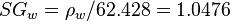$SG_w = \rho_w/62.428=1.0476$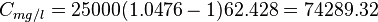$C_{mg/l} = 25000 (1.0476 - 1) 62.428 = 74289.32$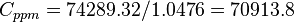$C_{ppm} = 74289.32 / 1.0476 = 70913.8$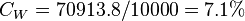$C_W = 70913.8 / 10000 = 7.1 %$

## Nomenclature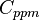$C_{ppm}$ = water salinity / solids concentration, ppm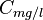$C_{mg/l}$ = water salinity / solids concentration, mg/l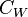$C_W$ = weight percent solids, %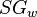$SG_w$ = water specific gravity, dimensionless

## See also

Water bubble point pressure
Water compressibility
Water density
Water formation volume factor
Water salinity from density equation
Water solids concentration
Water viscosity
Gas/Water Interfacial Tension